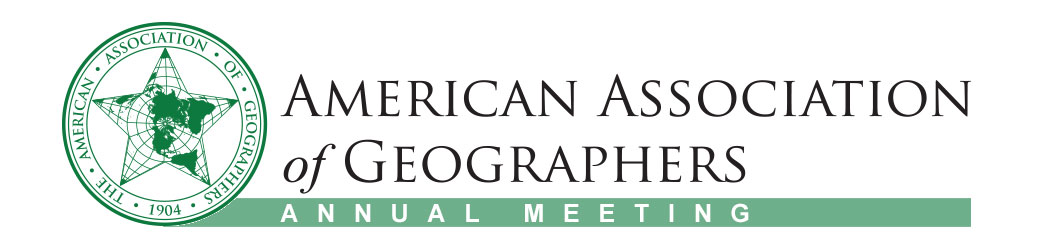#### A comparison of Markov Chain geostatistical simulation using linear interpolated and fitted transiogram models

Authors: Bo Zhang*, University of Connecticut, Weidong Li, University of Connecticut
Topics: Geographic Information Science and Systems
Keywords: Geostatistics, transiogram, Markov Chain
Session Type: Poster
Day: 4/4/2019
Start / End Time: 9:55 AM / 11:35 AM
Room: Lincoln 2, Marriott, Exhibition Level
Presentation File: No File Uploaded

Markov Chain Geostatistics (MCG) are a set of tools for modelling spatial data. The spatial measure of MCG is transiogram, which refers to a transition probability diagram. Transiogram provides the interclass and spatial relationship between categorical data. To get continuous transiograms from sample data, one can use linear interpolation or apply mathematical models to fit the experimental transiogram. In this study, we applied Markov Chain Geostatistics model to simulate the spatial distribution of surface soil variables. Two case studies are presented to show how optimal prediction maps would differ with different sets of transiogram models. Our results showed that MCG is a robust method for categorical variable modeling. The differences of simulation accuracies are not significant between applying linear interpolated transiogram and mathematical model fitted transiograms. One reason that could be used to explain the relative small difference in this study is that continuous transiograms from these two methods are both constrained by the experimental transiogram which is directly derived from observational data. Another reason is that the low lag part of continuous transiogram is fitted as closely to experimental transiogram as possible. The results of this study show that two methods of deriving continuous transiograms have their own advantages perform similarly in MCG simulation.

#### Abstract Information

This abstract is already part of a session. View the session here.

To access contact information login